SBOS826D December   2017  – October 2019

PRODUCTION DATA.

1. Features
2. Applications
3. Description
1.     Device Images
4. Revision History
5. Pin Configuration and Functions
6. Specifications
7. Detailed Description
1. 7.1 Overview
2. 7.2 Functional Block Diagram
3. 7.3 Feature Description
4. 7.4 Device Functional Modes
8. Application and Implementation
1. 8.1 Application Information
2. 8.2 Typical Applications
9. Power Supply Recommendations
10. 10Layout
11. 11Device and Documentation Support
1. 11.1 Device Support
1. 11.1.1 Development Support
2. 11.2 Documentation Support
4. 11.4 Support Resources
6. 11.6 Electrostatic Discharge Caution
12. 12Mechanical, Packaging, and Orderable Information

• D|8
• DGK|8
• DBV|5
• DGK|8

6.6 Typical Characteristics

at TA = 25°C, VS = ±15 V, and RL = 2 kΩ (unless otherwise noted)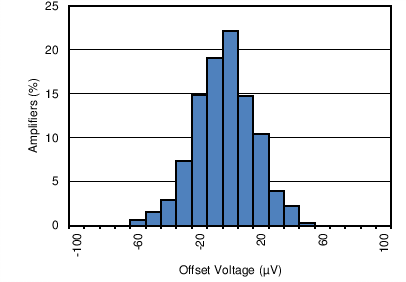Figure 1. Input Referred Offset Voltage Distribution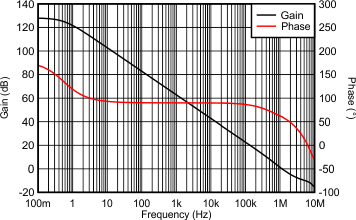Figure 3. Open-Loop Gain and Phase vs Frequency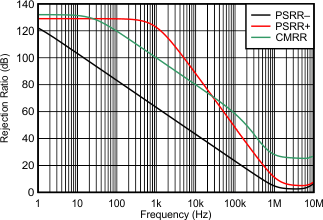Figure 5. Power Supply Rejection Ratio and Common-Mode Rejection Ratio vs Frequency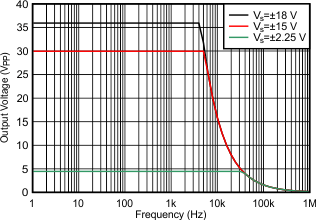Figure 7. Full Power BandwidthFigure 9. 0.1-Hz to 10-Hz Noise Voltage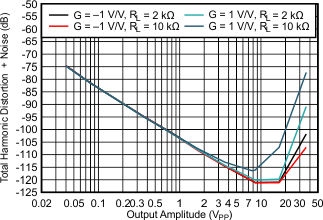Figure 11. Total Harmonic Distortion + Noise vs Output AmplitudeG = +1 V/V
Figure 13. Overshoot vs Capacitive Load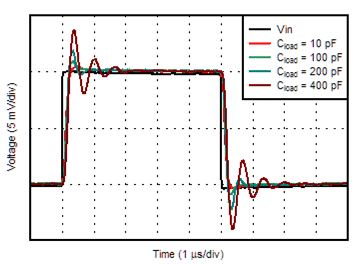G = +1 V/V
Figure 15. Small-Signal Step Response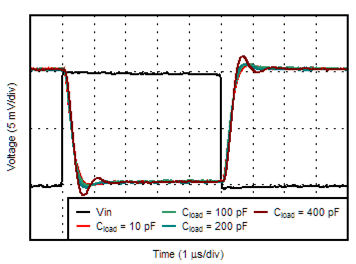G = –1 V/V
Figure 17. Small-Signal Step Response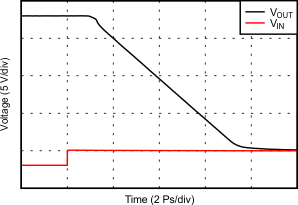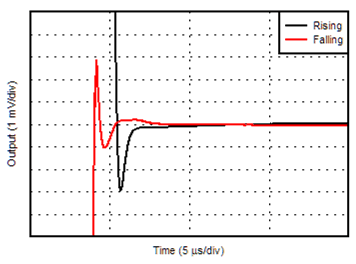VOUT = 3 VRMS
Figure 21. Settling Time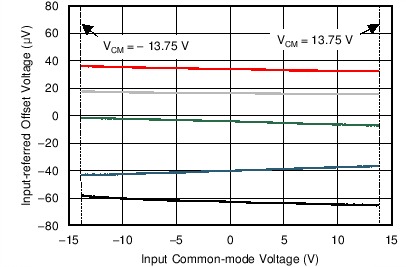Figure 23. Input Offset Voltage vs Input Common-mode VoltageFigure 25. Quiescent Current vs Power Supply Voltage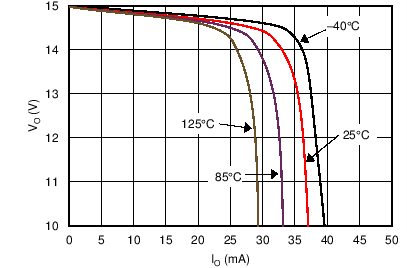Figure 27. Output Voltage vs Output Current (Sourcing)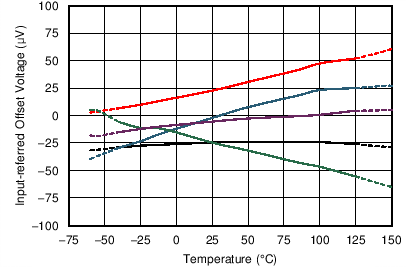Figure 29. Input Offset Voltage vs Temperature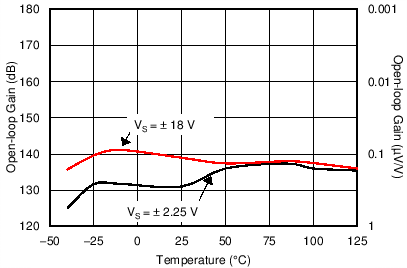Figure 31. Open Loop Gain vs TemperatureFigure 33. Power Supply Rejection Ratio vs Temperature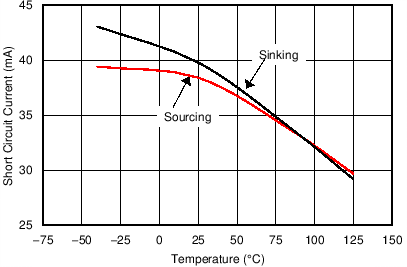Figure 35. Output Short Circuit Current vs TemperatureFigure 2. Input Referred Offset Voltage Drift Distribution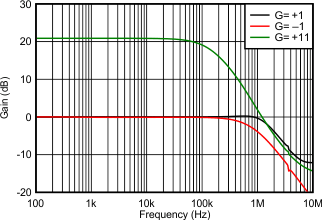Figure 4. Closed Loop Gain vs Frequency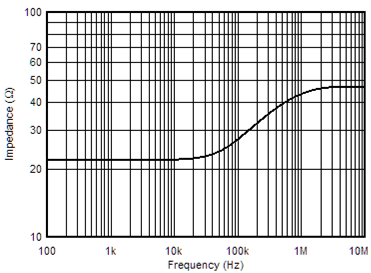Figure 6. Open-Loop Output Impedance vs Frequency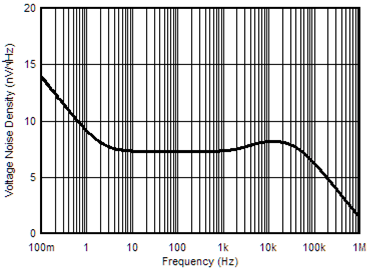Figure 8. Input Voltage Noise Spectral Density vs Frequency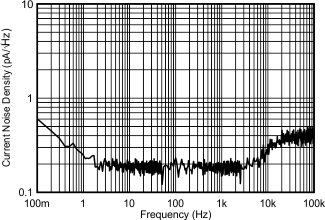Figure 10. Input Current Noise vs Frequency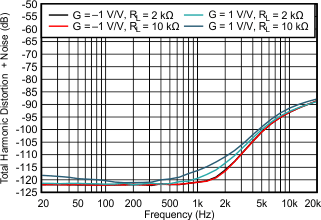VOUT = 3 VRMS
Figure 12. Total Harmonic Distortion + Noise vs Frequency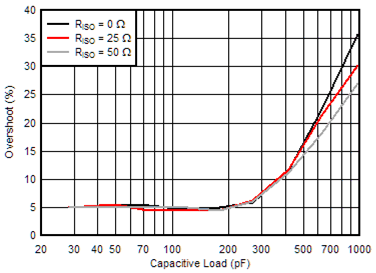G = –1 V/V
Figure 14. Overshoot vs Capacitive Load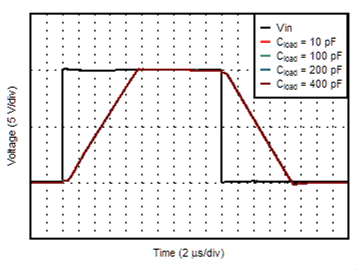G = +1 V/V
Figure 16. Large-Signal Step Response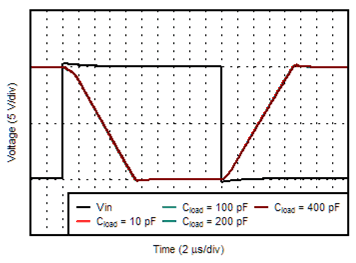G = –1 V/V
Figure 18. Large-Signal Step Response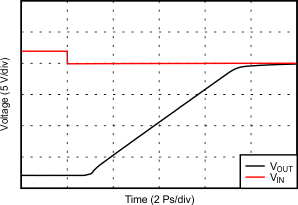VOUT = 3 VRMS
Figure 22. No Phase Reversal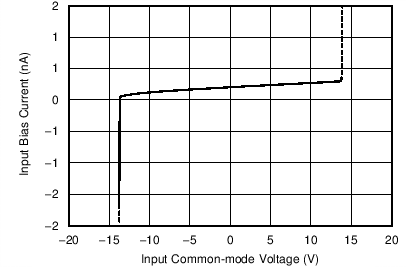Figure 24. Input Bias Current vs Input Common-mode VoltageFigure 26. Input Offset Voltage vs Power Supply Voltage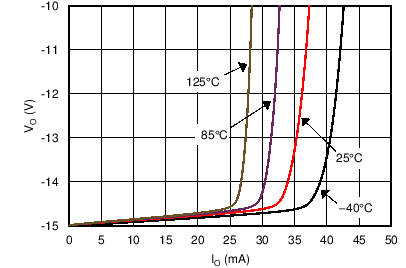Figure 28. Output Voltage vs Output Current (Sinking)Figure 30. Input Bias Current vs Temperature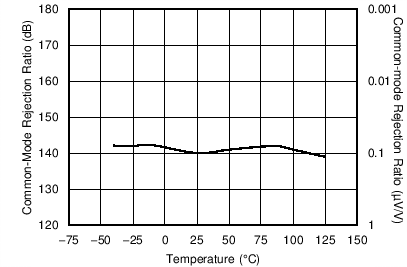Figure 32. Common-Mode Rejection Ratio vs Temperature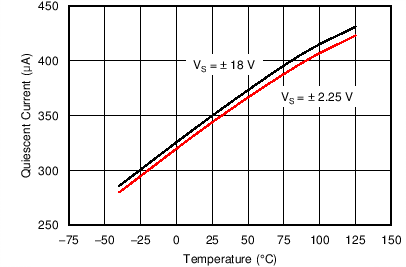Figure 34. Quiescent Current vs Temperature